# Schur Q-function

A symmetric function introduced by I. Schur [a6] in 1911 in the construction of the irreducible spin characters of the symmetric groups(cf. Projective representations of symmetric and alternating groups). Schur-functions are analogous to the Schur functions, which play the same role for linear characters (cf. Schur functions in algebraic combinatorics). In fact, both are special cases of Hall–Littlewood functions discovered by D.E. Littlewood [a3], but see [a4] for a description of their development and subsequent generalizations, for example, Macdonald polynomials.

There are by now (as of 2000) several other definitions; the original by Schur [a6] was in terms of Pfaffians (cf. Pfaffian), a modern version of his work is [a2]. Letbe a set of variables (); then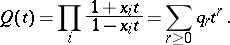(a1)

For, defineand then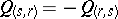, because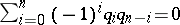, as follows directly from (a1).

If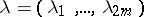is a strict partition of, where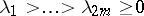, then the matrix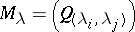is skew-symmetric, and the Schur-function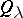is defined aswhere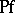stands for the Pfaffian.

An alternative purely combinatorial definition has been given by J.R. Stembridge [a7] in terms of shifted (Young) diagrams. These differ from Young diagrams (cf. Young diagram) in that there is an indentation along the diagonal. Young tableaux are replaced by marked shifted tableaux which are defined as follows. Letdenote the ordered alphabet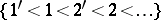; then a marked shifted tableauis a labelling of the nodes of the shifted diagram of shapesuch that

i) the labels weakly increase along each row and down each column;

ii) each column contains at most one, for each; and

iii) each row contains at most one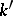, for each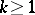. If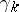denotes the number of nodes labelled eitheror, then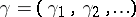is the content ofand if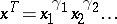, then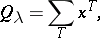summed over all marked shifted tableaux of shape. It is a non-trivial task to prove that this is the Schur-function. For example, if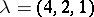, then the corresponding shifted diagram and a possible marked shifted tableau are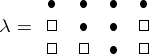and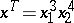.

This combinatorial definition has been a rich source of significant combinatorial results, for example, Stembridge [a7] has proved an analogue of the Littlewood–Richardson rule that describes the Schur-function expansion of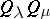and also gives a purely combinatorial proof for the Murnaghan–Nakayama rule for computing the irreducible spin characters of(cf. Representation of the symmetric groups). All of this is based on a shifted version of the Robinson–Schensted–Knuth correspondence given independently by B.E. Sagan [a5] and D.R. Rowley (cf. also Robinson–Schensted correspondence).

Schur-functions also arise naturally in other contexts, for example, the characters of irreducible representations of the queer Lie super-algebra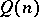, the cohomology classes dual to Schubert cycles in isotropic Grassmannians and in polynomial solutions of the BKP-hierarchy of partial differential equations.